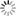# Episode List

OR

### Season 1

14 Nov. 1956
Bardell vs. PickwickBe the first one to add a plot.

19 Oct. 1956
The Brown Man's ServantBe the first one to add a plot.

9 Oct. 1955
The DoorBe the first one to add a plot.

26 Dec. 1956
The Great HealerIn Victorian times, two women meet up, it comes out that they hadn't seen each other for over fifty years, from when they worked together as chorus girls. They have a chat about old times and how their lives have changed, the most notable changes are Kitty's who went from been a working class chorus girl to a titled lady after marring Bertie, who would later become Lord Bodmin. Rosie's changes were more mundane as she just married and became Mrs. Bounce.

28 Nov. 1956
Mister Betts Runs AwayBe the first one to add a plot.

30 Oct. 1955
Mossbach CollectionBe the first one to add a plot.

6 Nov. 1955
The OrderlyBe the first one to add a plot.

5 Dec. 1956
The Little Black BookBe the first one to add a plot.

12 Dec. 1956
The Suicide ClubBe the first one to add a plot.

28 Sep. 1956
The Game and the OnlookerBe the first one to add a plot.

4 Dec. 1955
Death TrapBe the first one to add a plot.

21 Nov. 1956
The No ManBe the first one to add a plot.

18 Dec. 1955
The StockingBe the first one to add a plot.

25 Dec. 1955
A Present from BessieBe the first one to add a plot.

1 Jan. 1956
The TriumphantBe the first one to add a plot.

8 Jan. 1956
The Stolen PearlBe the first one to add a plot.

15 Jan. 1956
The ColonelBe the first one to add a plot.

26 Oct. 1956
Bride Wore an Opal RingBe the first one to add a plot.

21 Sep. 1956
The AssassinBe the first one to add a plot.

5 Feb. 1956Be the first one to add a plot.

2 Nov. 1956
The PortraitBe the first one to add a plot.

19 Feb. 1956
The Old Man of the AirBe the first one to add a plot.

26 Feb. 1956
The Case of Dr. CrippenDr. Crippen is accused of murdering his nymphomaniac wife.

4 Mar. 1956
Night CallBe the first one to add a plot.

11 Mar. 1956Be the first one to add a plot.

12 Oct. 1956
Death Under the CityBe the first one to add a plot.

5 Oct. 1956
The Weakness of Frau BorkhardtBe the first one to add a plot.

1 Apr. 1956
The LovebirdsBe the first one to add a plot.

8 Apr. 1956
On Any One DayBe the first one to add a plot.

15 Apr. 1956Be the first one to add a plot.

22 Apr. 1956
The GlovesBe the first one to add a plot.

19 Dec. 1956
The Ends of JusticeBe the first one to add a plot.

6 May 1956
Forecast UnsettledBe the first one to add a plot.

13 May 1956
Episode in Paris